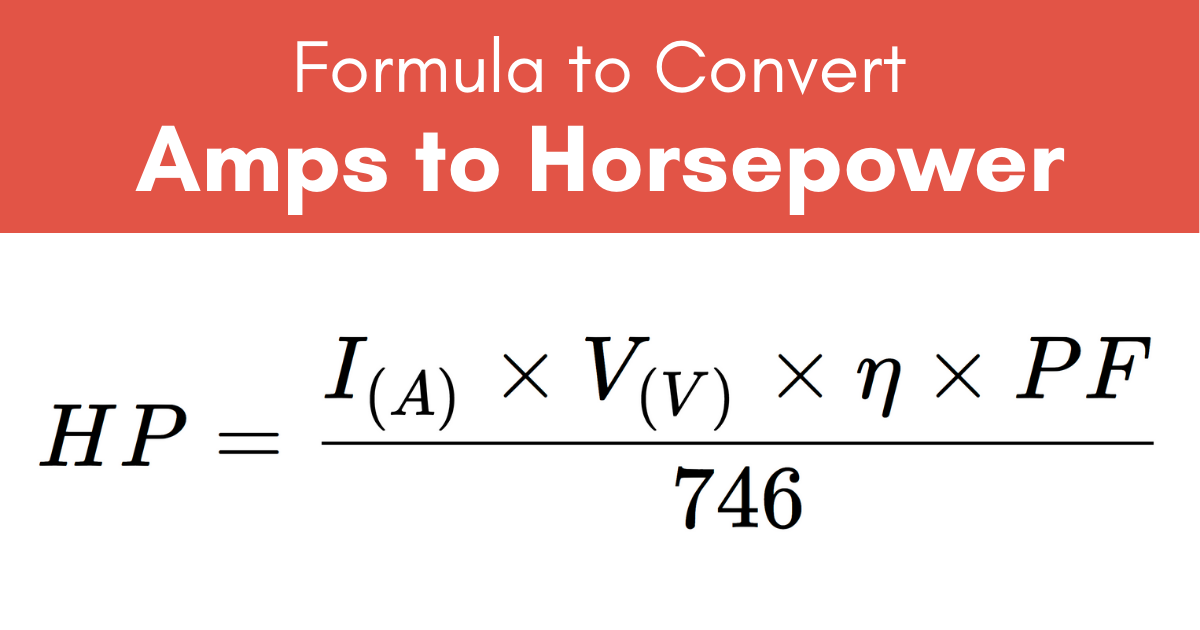# Amps to Horsepower Calculator

Enter amps, volts, and motor efficiency to calculate the horsepower.

amps
volts
%

## Power:

Horsepower
Learn how we calculated this below

## How to Convert Amps to Horsepower

The conversion from amps, a measure of electric current, to horsepower, a measure of power output by a motor, can be done using a fairly simple formula.

P(HP) = I(A) × V(V) × η × PF / 746

Thus, the power P in horsepower is equal to the current I in amps times the voltage V in volts times the efficiency η times the power factor PF, divided by 746.How did we get that? This formula can be derived in two steps.

### Step One: Calculate the Power

Since horsepower is a measure of electric power, Watt’s Law can be used to convert. Watt’s Law gives us the formula to solve power given voltage, current, and AC power factor using this formula.

P(W) = I(A) × V(V) × PF

The formula states that the power P in watts is equal to the current I in amps times the voltage V in volts times the power factor PF.

One additional consideration that should be made when doing the conversion is the efficiency of the motor being used. Motors are often only 80% to 90% efficient, which means that they can only produce 80% to 90% of energy output for the amount of power in.

When converting to horsepower, the efficiency can be accounted for by multiplying the result by the efficiency η in decimal form.

P(W) = I(A) × V(V) × PF× η

### Step Two: Convert the Power to Horsepower

After converting amps and volts to watts, the next step is to convert watts to horsepower. The table below offers a quick reference for how to convert watts to electrical or mechanical horsepower.

Conversion of watts to various types of horsepower.
Horsepower Watts
1 Electric HP 746 watts
1 Mechanical HP 745.7 watts
1 Metric HP 735.5 watts

Because 1 horsepower is equal to 746 watts, the formula above will include a division by 746 to complete the conversion.

P(HP) = P(W) / 746 WHP

For example, let’s find the horsepower of a motor drawing 8 amps at 120 volts that is 90% efficient. Assume a PF of 1.

P(HP) = 8 A × 120 V × 0.9 × 1 / 746 WHP
P(HP) = 864 W / 746 WHP
P(HP) = 1.158 HP

## Common Amps to Horsepower Conversions

Power measurements in horsepower for common amperage values at 120 volts for a motor with 90% efficiency and power factor of 1.
Amps Horsepower Voltage Efficiency
1 A .14 HP 120 V 90%
2 A .29 HP 120 V 90%
3 A .43 HP 120 V 90%
4 A .58 HP 120 V 90%
5 A .72 HP 120 V 90%
6 A .87 HP 120 V 90%
7 A 1.01 HP 120 V 90%
8 A 1.16 HP 120 V 90%
9 A 1.3 HP 120 V 90%
10 A 1.45 HP 120 V 90%
15 A 2.17 HP 120 V 90%
20 A 2.9 HP 120 V 90%
25 A 3.62 HP 120 V 90%
30 A 4.34 HP 120 V 90%

We also have a horsepower to amps calculator that you may find useful.

## References

1. Miller, C., NFPA's Electrical References, National Fire Protection Association, 2004, Jones & Bartlett Learning, 67-75. https://www.google.com/books/edition/NFPA_s_Electrical_References/raUyIi7i-asC